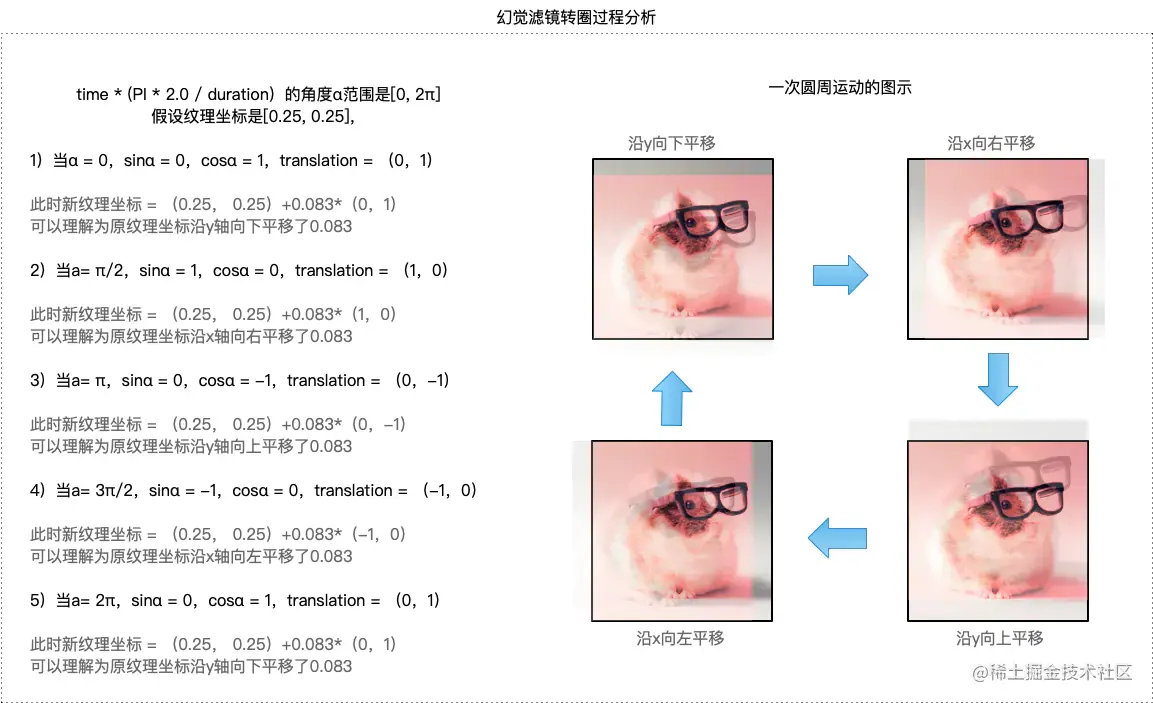# OpenGL-ES-案例13：动效滤镜(6种)

## 滤镜算法

6种滤镜的思路及实现如下所示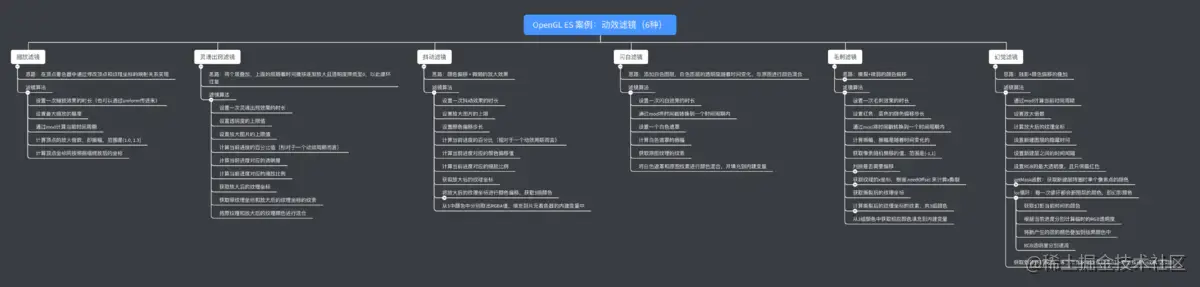### 缩放滤镜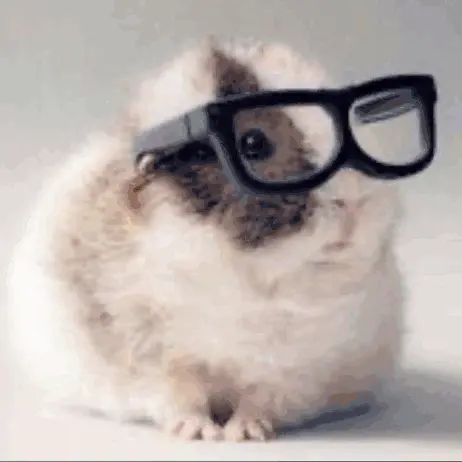• 通过mod取模函数计算当前时间戳对应的时间周期

• 计算顶点的放大倍数，即缩放的振幅，范围是[1.0, 1.3]

• 计算顶点坐标按照振幅缩放后的坐标 如下图所示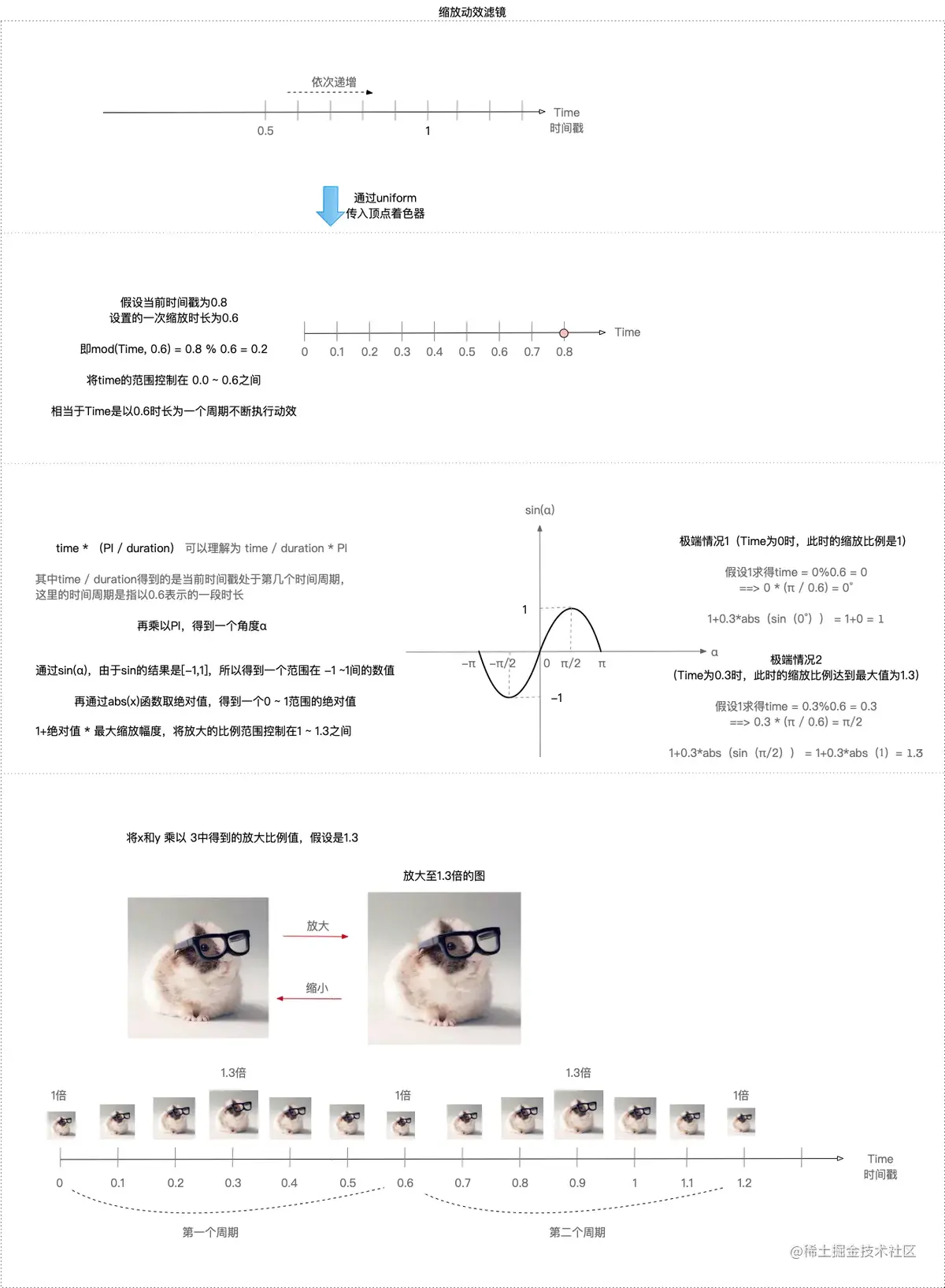``````attribute vec4 Position;
attribute vec2 TextureCoords;
varying vec2 TextureCoordsVarying;
//时间戳（随着定时器的方法调用及时更新）:从0开始一直递增
uniform float Time;
const float PI = 3.1415926;

void main(){
//一次缩放效果的时长
float duration = 0.6;
//最大缩放幅度
float maxAmplitude = 0.3;

//表示传入的事件周期，即time的范围被控制在0.0~0.6
//mod(a, b)，求模运算 等价于 a%b，GLSL中不支持%求模
float time = mod(Time,duration);

//amplitude表示振幅，引入PI的目的是为了使用sin函数，将amplitude的范围控制在1.0 ~ 1.3之间，并随着时间变化
//这里可以不用取绝对值，因为角度的范围是【0，π】，不会出现负数的情况
float amplitude = 1.0 + maxAmplitude * abs(sin(time * (PI / duration)));

//放大关键代码：将顶点坐标的x和y分别乘以一个放大系数，即振幅，在纹理坐标不变的情况下，就达到了拉伸的效果
//xy放大，zw保持不变
gl_Position = vec4(Position.x * amplitude, Position.y * amplitude, Position.zw);

//纹理坐标传递给TextureCoordsVarying
TextureCoordsVarying = TextureCoords;
}

### 灵魂出窍滤镜• 根据当前时间戳计算当前周期在一个周期时长中的百分比，即进度
• 计算当前进度对应的透明度
• 计算当前进度对应的缩放比例
• 获取放大后的纹理坐标
• 获取原纹理坐标 & 放大纹理坐标的纹素
• 将原纹理和放大后的纹理进行颜色混合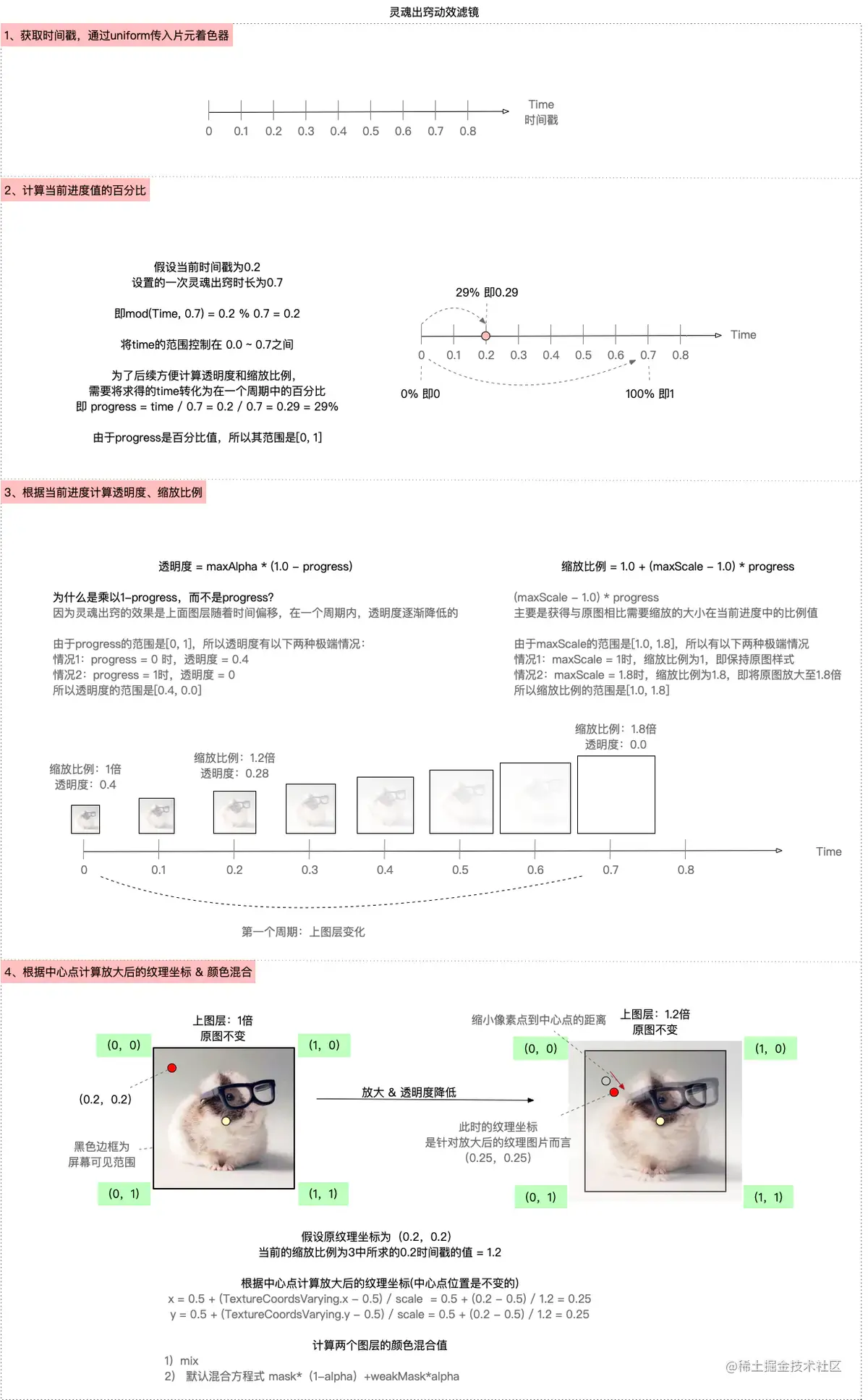``````precision highp float;

uniform sampler2D Texture;
varying vec2 TextureCoordsVarying;
//时间戳
uniform float Time;

void main (void) {
//一次灵魂出窍效果的时长
float duration = 0.7;
//透明度上限值
float maxAlpha = 0.4;
//图片放大的上限
float maxScale = 1.8;

//当前进度（时间戳与时长使用mod取模）,再除以时长 得到【0， 1】，即百分比
float progress = mod(Time, duration) / duration; // 0~1
//当前透明度 【0.4， 0】
float alpha = maxAlpha * (1.0 - progress);
//当前缩放比例 【1.0， 1.8】
float scale = 1.0 + (maxScale - 1.0) * progress;

//获取放大后的纹理坐标
//将顶点坐标对应的纹理坐标的x/y值到中心点的距离，缩小一定的比例，仅仅只是改变了纹理坐标，而保持顶点坐标不变，从而达到拉伸效果
float weakX = 0.5 + (TextureCoordsVarying.x - 0.5) / scale;
float weakY = 0.5 + (TextureCoordsVarying.y - 0.5) / scale;
vec2 weakTextureCoords = vec2(weakX, weakY);

//获取当前像素点纹理坐标，放大后的纹理坐标

//2、颜色混合 内建函数mix / 混合方程式
}

### 抖音抖动滤镜• 根据当前时间戳计算当前进度的百分比
• 计算当前进度对应的颜色偏移
• 计算当前进度对应的缩放比例
• 获取放大后的纹理坐标
• 将放大后的纹理纹素进行颜色偏移，获得3组颜色
• 从3组颜色中分别获取RGBA的值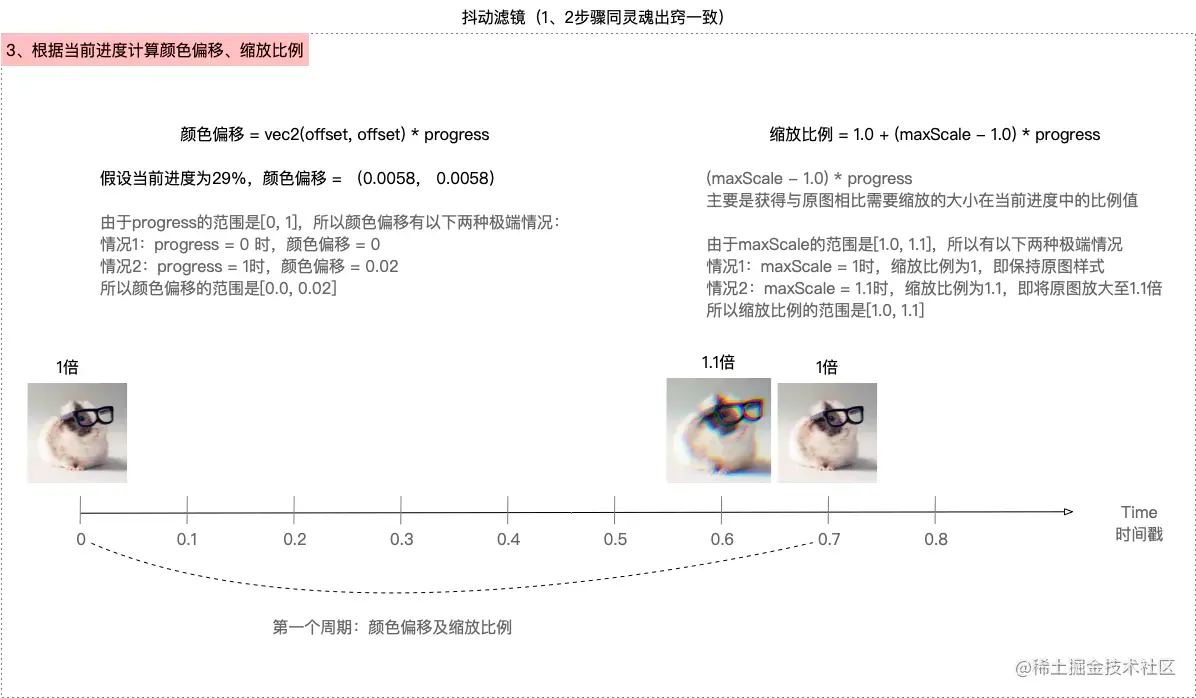``````void main(){
//一次抖动效果的时长
float duration = 0.7;
//放大图片的上限
float maxScale = 1.1;
//颜色偏移的步长
float offset = 0.02;

//进度 0 ~ 1
float progress = mod(Time, duration) / duration;
//颜色偏移值0 ~ 0.02
vec2 offsetCoords = vec2(offset, offset) * progress;
//缩放比例 1.0 ~ 1.1
float scale = 1.0 + (maxScale - 1.0) * progress;

//放大后的纹理坐标
//下面这种向量相加减的方式 等价于 灵魂出窍滤镜中的单个计算x、y坐标再组合的为纹理坐标的方式
vec2 ScaleTextureCoords = vec2(0.5, 0.5) + (TextureCoordsVarying - vec2(0.5, 0.5)) / scale;

//获取三组颜色：颜色偏移计算可以随意，只要偏移量很小即可
//原始颜色 + offset
vec4 maskR = texture2D(Texture, ScaleTextureCoords + offsetCoords);
//原始颜色 - offset
vec4 maskB = texture2D(Texture, ScaleTextureCoords - offsetCoords);
//原始颜色

//从3组颜色中分别取出 红色R，绿色G，蓝色B，透明度A填充到内置变量gl_FragColor内
}

### 闪白滤镜• 通过mod函数计算当前时间戳对应的时间周期
• 设置一个白色遮罩
• 计算白色遮罩的振幅，振幅范围是[0,0, 1.0]
• 获取原图纹理的纹素，并与白色遮罩颜色混合 颜色混合的方式有多种，常用的一般是`mix函数`或者`默认的混合方程式：mask*(1-alpha + weakMask*alpha`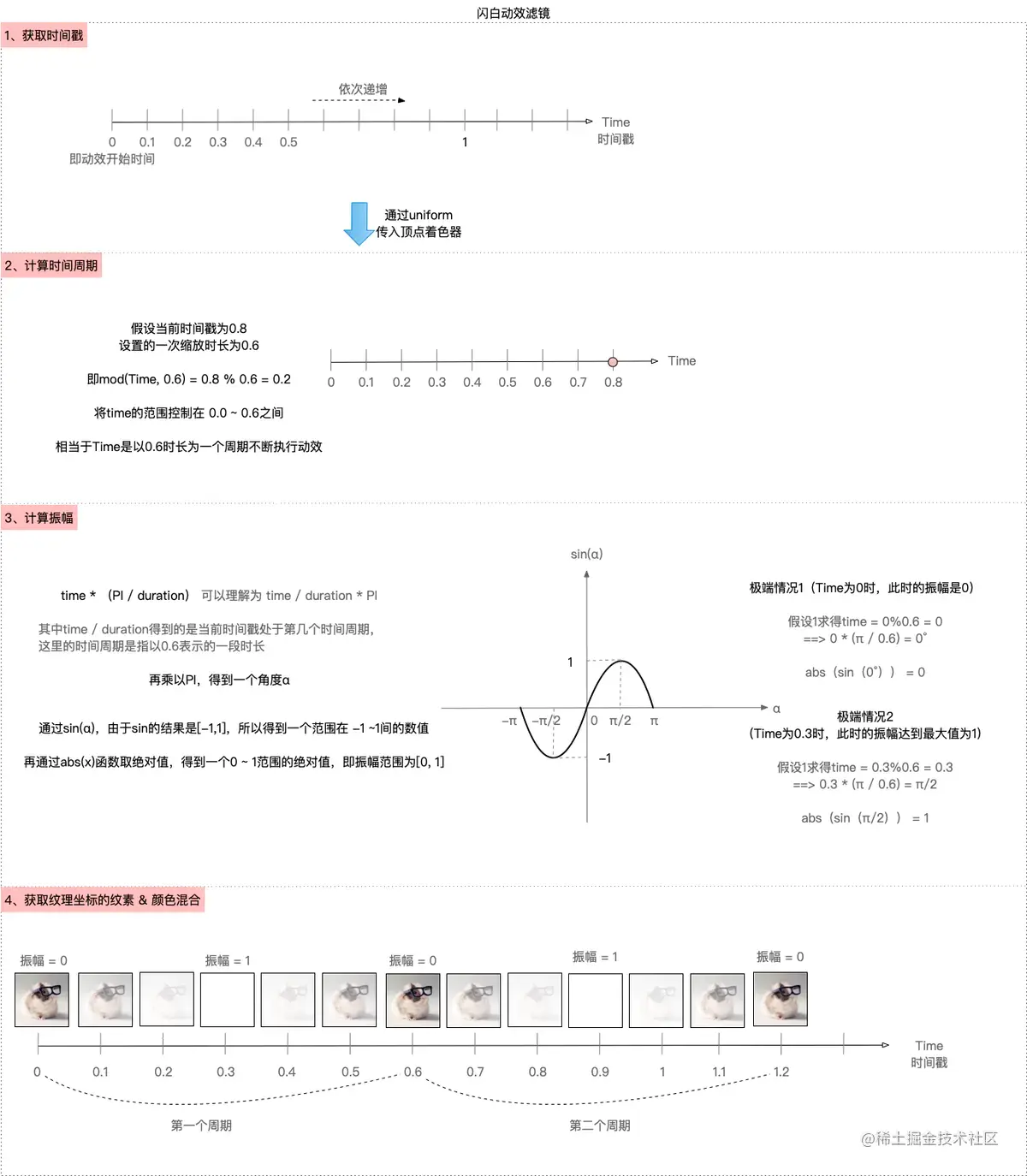``````precision highp float;
uniform sampler2D Texture;
varying vec2 TextureCoordsVarying;
//时间戳
uniform float Time;
//PI常量
const float PI = 3.1415926;

void main(){
//一次闪白的时长
float duration = 0.6;
//将时间戳转换到一个周期内，范围是0 ~ 0.6
float time = mod(Time, duration);
//白色遮罩
vec4 whiteMask = vec4(1.0, 1.0, 1.0, 1.0);
// 振幅，范围是0 ~ 1
//    float amplitude = abs(sin(time * (PI / duration)));
float amplitude = sin(time * (PI / duration));

//获取纹理坐标对应的纹素颜色值
//利用mix内置函数进行颜色混合，属于线性混合
}

### 毛刺滤镜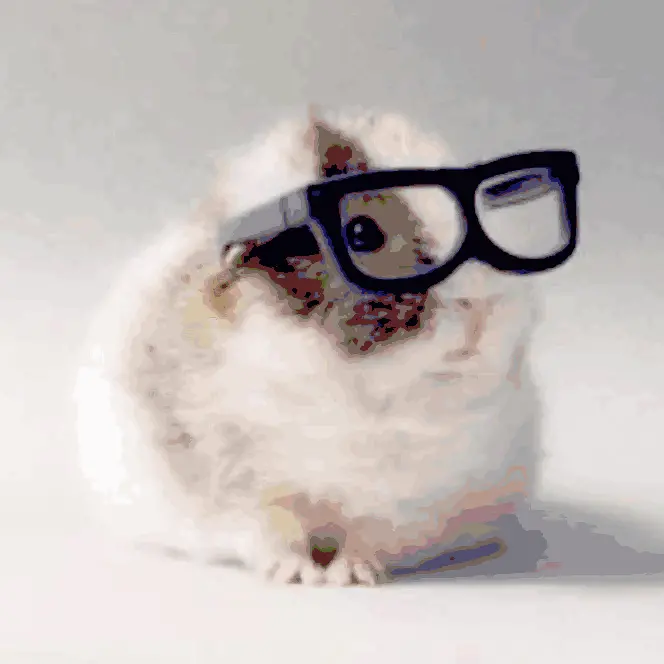• mod函数计算时间周期
• 计算振幅，范围是「0, 1]
• 获取像素点随机偏移值，范围是[-1,1]
• 判断是否需要偏移 & 计算纹理的x坐标
• 需要偏移，撕裂较大，即x的颜色偏移较大
• 不需要，撕裂较小，即x的颜色偏移值很微小
• 获取撕裂后的纹理坐标
• 计算撕裂后的3组纹素，并获取不同组中的RGBA值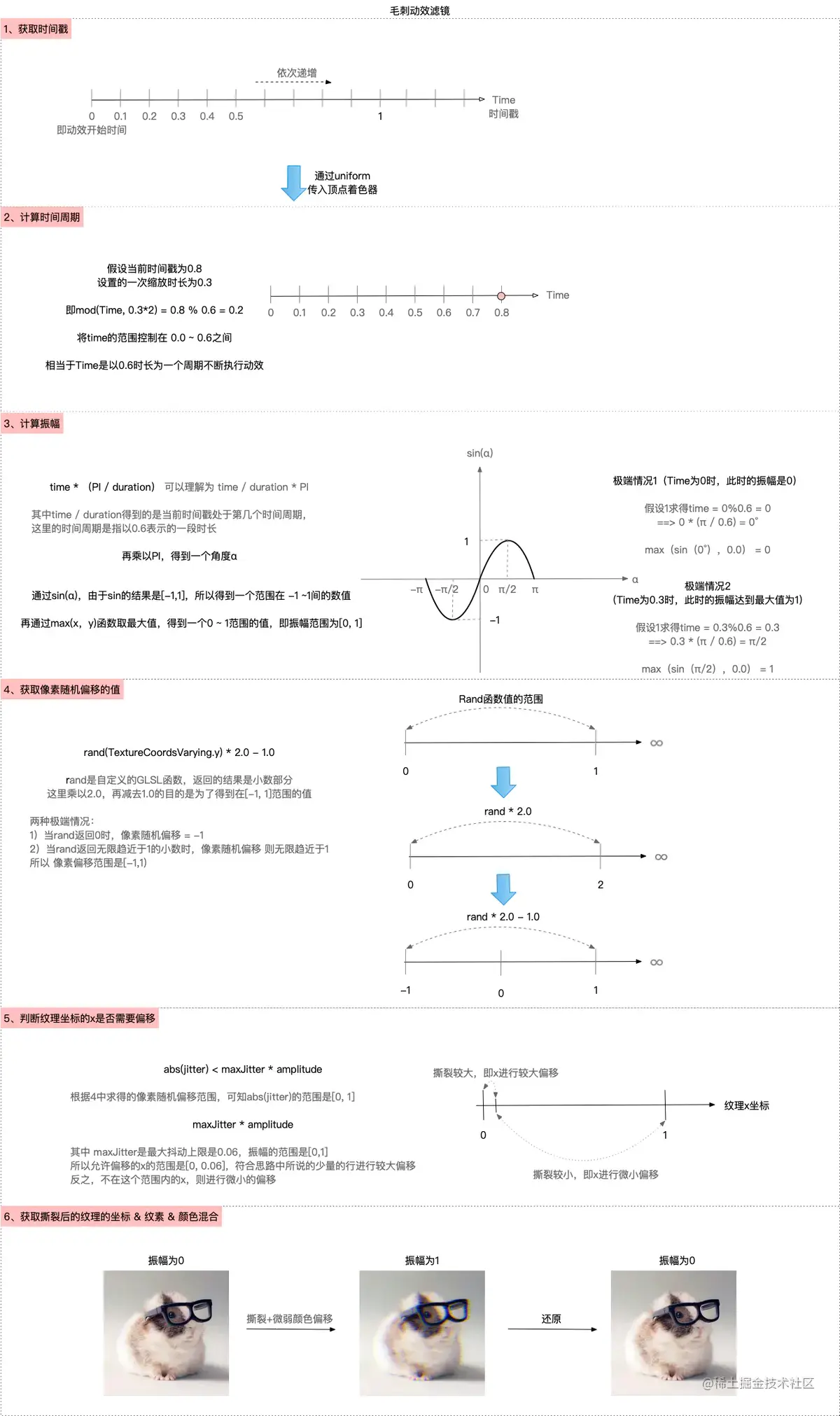``````precision highp float;
uniform sampler2D Texture;
varying vec2 TextureCoordsVarying;
//时间戳
uniform float Time;
//PI常量
const float PI = 3.1415926;
//随机数
float rand(float n){
//fract(x)返回x的小数部分
//返回 sin(n) * 43758.5453123
//sin(n) * 极大值，带小数点，想要随机数算的比较低，乘的数就必须较大，噪声随机
//如果想得到【0，1】范围的小数值，可以将sin * 1
//如果只保留小数部分，乘以一个极大值
return fract(sin(n) * 43758.5453123);
}

void main(){
//最大抖动上限
float maxJitter = 0.06;
//一次毛刺效果的时长
float duration = 0.3;
//红色颜色偏移
float colorROffset = 0.01;
//绿色颜色偏移
float colorBOffset = -0.025;

//表示将传入的事件转换到一个周期内，范围是 0 ~ 0.6，抖动时长变成0.6
float time = mod(Time, duration * 2.0);
//振幅，随着时间变化，范围是[0, 1]
float amplitude = max(sin(time * (PI / duration)), 0.0);

//像素随机偏移范围 -1 ~ 1，* 2.0 - 1.0是为了得到【-1，1】范围内的随机值
float jitter = rand(TextureCoordsVarying.y) * 2.0 - 1.0;
//判断是否需要偏移，如果jitter范围 < 最大范围*振幅
// abs(jitter) 范围【0，1】
// maxJitter * amplitude 范围【0， 0.06】
bool needOffset = abs(jitter) < maxJitter * amplitude;

//获取纹理x坐标，根据needOffset来计算它的x撕裂
//needOffset = YES，则撕裂大
//needOffset = NO，则撕裂小，需要降低撕裂 = *振幅*非常细微的数
float textureX = TextureCoordsVarying.x + (needOffset ? jitter : (jitter * amplitude * 0.006));
//获取纹理撕裂后的x、y坐标
vec2 textureCoords = vec2(textureX, TextureCoordsVarying.y);

//颜色偏移：获取3组颜色
//撕裂后的原图颜色
//根据撕裂计算后的纹理坐标，获取纹素
vec4 maskR = texture2D(Texture, textureCoords + vec2(colorROffset * amplitude, 0.0));
//根据撕裂计算后的纹理坐标，获取纹素
vec4 maskB = texture2D(Texture, textureCoords + vec2(colorBOffset * amplitude, 0.0));

//颜色主要撕裂，红色和蓝色部分，所以只调整红色
}

GLSL中没有随机值的函数，需要通过自定义函数，利用fract函数获得随机数

### 幻觉滤镜

• 残影：是每隔一段时间，就会新建一个图层，且该图层以红色为主，随着时间推移透明度逐渐降低，于是可以在一个周期时长内看到很多不同透明度的层叠加在一起，从而形成残影，让图片随着时间做圆周运动
• 颜色偏移：图片在移动的过程中是蓝色在前，红色在后，即在移动的过程中，每间隔一段时间，遗失了一部分红色通道的值在原来的位置，并且这部分红色通道的值，随着时间偏移，会逐渐恢复。• 通过mod函数计算当前时间周期
• 设置放大倍数
• 计算放大后的纹理坐标
• 获取转全过程中像素点的纹素
• 通过for循环来新建图层，即幻影颜色
• 获取由原始图层和新建层叠加的颜色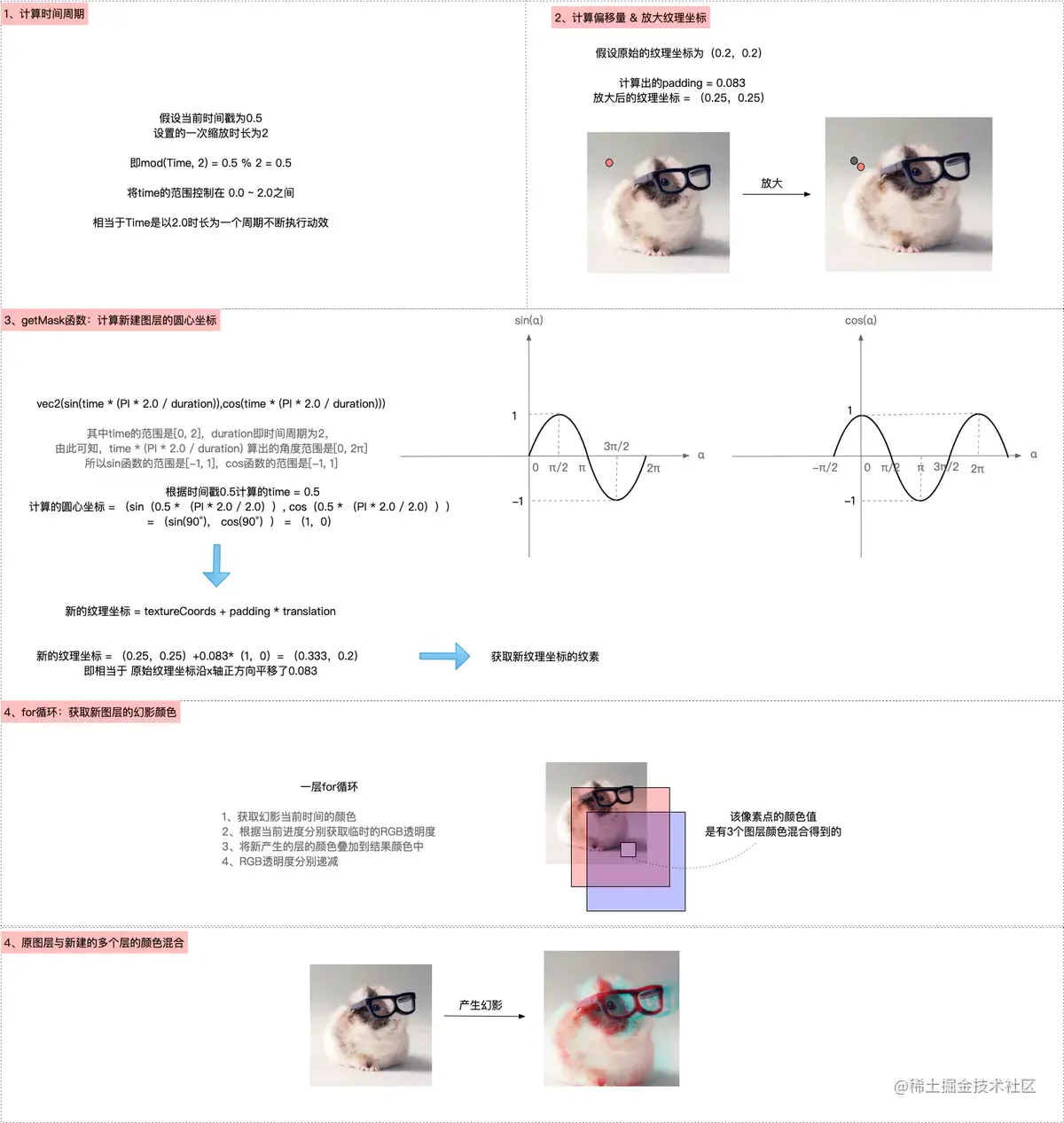``````precision highp float;
uniform sampler2D Texture;
varying vec2 TextureCoordsVarying;

uniform float Time;

const float PI = 3.1415926;
//一次幻觉效果的时长，即周期
const float duration = 2.0;

//转圈产生幻影的单个像素点的颜色值
//圆心坐标
vec2 translation = vec2(sin(time * (PI * 2.0 / duration)),cos(time * (PI * 2.0 / duration)));

//新的纹理坐标 = 原始纹理坐标 + 偏移量 * 圆周坐标（新的图层与图层之间是有间距的，所以需要偏移）
vec2 translationTextureCoords = textureCoords + padding * translation;

//根据新的纹理坐标获取新图层的纹素

}

//这个函数可以计算出，某个时刻创建的层，在当前时刻的透明度
//进度：
float maskAlphaProgress(float currentTime, float hideTime, float startTime) {
//mod（时长+持续时间 - 开始时间，时长）得到一个周期内的time
float time = mod(duration + currentTime - startTime, duration);
//如果小于0.9，返回time，反之，返回0.9
return min(time, hideTime);
}

void main(){
//将传入的时间戳转换到一个周期内，time的范围是【0，2】
//获得时间周期
float time = mod(Time, duration);
//放大后的倍数
float scale = 1.2;
//偏移量 = 0.083
float padding = 0.5 * (1.0 - 1.0 / scale);
//放大后的纹理坐标
vec2 textureCoords = vec2(0.5, 0.5) + (TextureCoordsVarying - vec2(0.5, 0.5)) / scale;

//新建层的隐藏时间 即新建层什么时候隐藏
float hideTime = 0.9;
//时间间隔：隔0.2s创建一个新层
float timeGap = 0.2;

//注意：只保留了红色的透明的通道值，因为幻觉效果残留红色
//幻影残留数据
//max RGB alpha
//新图层的 R透明度
float maxAlphaR = 0.5;
//新图层的 G透明度
float maxAlphaG = 0.05;
//新图层的 B透明度
float maxAlphaB = 0.05;

//获取新的图层的坐标，需要传入时间、纹理坐标、偏移量
//RGB ：for循环中使用
float alphaR = 1.0;
float alphaG = 1.0;
float alphaB = 1.0;

//最终图层颜色：初始化
vec4 resultMask = vec4(0, 0, 0, 0);

//循环：每一层循环都会得到新的图层的颜色，即幻影颜色
//一次循环只是计算一个像素点的纹素，需要在真机运行。模拟器会卡，主要是模拟器上是CPU模拟GPU的
for (float f = 0.0; f < duration; f += timeGap) {
float tmpTime = f;
//获取到【0，2】s内所获取的运动后的纹理坐标
//获得幻影当前时间的颜色值

//某个时刻创建的层，在当前时刻的红绿蓝的透明度
//临时的透明度 = 根据时间推移RGB的透明度发生变化
//获得临时的红绿蓝透明度
float tmpAlphaR = maxAlphaR - maxAlphaR * maskAlphaProgress(time, hideTime, tmpTime) / hideTime;
float tmpAlphaG = maxAlphaG - maxAlphaG * maskAlphaProgress(time, hideTime, tmpTime) / hideTime;
float tmpAlphaB = maxAlphaB - maxAlphaB * maskAlphaProgress(time, hideTime, tmpTime) / hideTime;

//累计每一层临时RGB * RGB的临时透明度
//结果 += 临时颜色 * 透明度，即刚产生的图层的颜色

//透明度递减
alphaR -= tmpAlphaR;
alphaG -= tmpAlphaG;
alphaB -= tmpAlphaB;
}

//最终颜色 += 原始纹理的RGB * 透明度